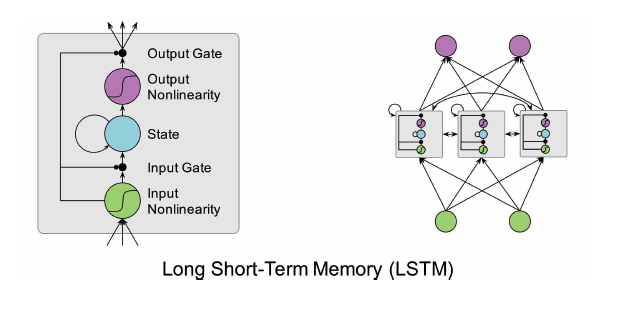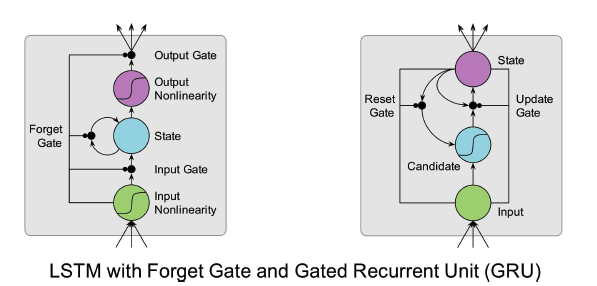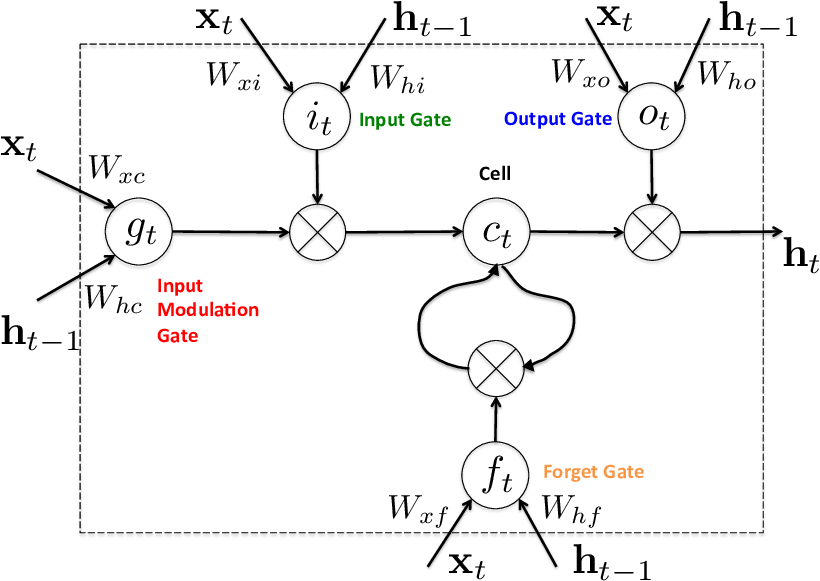# LSTM-Long Short Term Memory

LSTM it a type of Recurrent neural network, Various variants of RNNs have been around since the 1980s but were not widely used until recently because of insufficient computation power and difficulties in training. Since the invention of architectures like LSTM in 2006 we have seen very powerful applications of RNNs.

In 1997 by Hochreiter & Schmidhuber, LSTM is a special form of RNN that is designed to overcome the vanishing and exploding gradient problem. It works significantly better for learning long-term dependencies and has become a defactostandard for RNNs. In order to cope with the problem of vanishing and exploding gradients, the LSTM architecture replaces the normal neurons in an RNN with so-called LSTM cells that have a little memory inside. Those cells are wired together as they are in a usual RNN but they have an internal state that helps to remember errors over many time steps.

In the original LSTM cell, there are two gates: One learns to scale the incoming activation and one learns to scale the outgoing activation. The cell can thus learn when to incorporate or ignore new inputs and when to release the feature it represents to other cells. The input to a cell is feeded into all gates using individuals weights.A popular extension to LSTM is to add a forget gate scaling the internal recurrent connection, allowing the network to learn to forget (Gers, Felix A., Jürgen Schmidhuber, and Fred Cummins. “Learning to forget: Continual prediction with LSTM.” Neural computation 12.10 (2000): 2451-2471.). The derivative of the internal recurrent connection is now the activation of the forget gate and can differ from the value of one. The network can still learn to leave the forget gate closed as long as remembering the cell context is important.

It is important to initialize the forget gate to a value of one so that the cell starts in a remembering state. Forget gates are the default is almost all implementations nowadays. In TensorFlow, we can initialize the bias values of the forget gates by specifying the forget_bias parameter to the LSTM layer. The default is the value one and usually its best to leave it that wayAnother extension are so called peephole connections, which allows the gates to look at the cell state (Gers, Felix A., Nicol N. Schraudolph, and Jürgen Schmidhuber. “Learning precise timing with LSTM recurrent networks.” The Journal of Machine Learning Research 3 (2003): 115-143.). The authors claim that peephole connections are benefitial when the task involves precise timing and intervals. TensorFlow’s LSTM layer supports peephole connections. They can be activated by passing the use_peepholes=True flag to the LSTM layer.

Based on the idea of LSTM, an alternative memory cell called Gated Recurrent Unit (GRU) has been proposed in 2014 (Chung, Junyoung, et al. “Empirical evaluation of gated recurrent neural networks on sequence modeling.” arXiv preprint arXiv:1412.3555 (2014).). In contrast to LSTM, GRU has a simpler architecture and requires less computation while yielding very similar results. GRU has no output gate and combines the input and forget gates into a single update gate.

There are a few distinctions:

• GRU has 2 gates: update gate 𝑧 and reset gate 𝑟; LSTM has 3: input 𝑖, forget 𝑓, and output 𝑜
• LSTM maintains an internal memory state 𝐜, while GRU doesn’t
• LSTM applies a nonlinearity (sigmoid) before the output gate, GRU doesn’t

In both cases, each gate will have its own recurrent weight 𝐖 and input weight 𝐔. For example, the formula for input gate on LSTM is: 𝑖=𝜎(𝑥𝑡𝐔𝑖+𝑠𝑡1𝐖𝑖). It follows that GRUs have less parameters (weights) due to fewer number of gates and more efficient to train.

Bidirectional RNN: A Bidirectional RNN has composed of two RNNs that process data in opposite directions. One reads a given sequence from start to finish; the other reads it from finish to start. Bidirectional RNNs are employed in NLP for translation problems, among other use cases.

Gates in LSTM:In Keras we have folllowing class available,

keras.layers.LSTM(units, activation='tanh', recurrent_activation='hard_sigmoid', use_bias=True, kernel_initializer='glorot_uniform', recurrent_initializer='orthogonal', bias_initializer='zeros', unit_forget_bias=True, kernel_regularizer=None, recurrent_regularizer=None, bias_regularizer=None, activity_regularizer=None, kernel_constraint=None, recurrent_constraint=None, bias_constraint=None, dropout=0.0, recurrent_dropout=0.0, implementation=1, return_sequences=False, return_state=False, go_backwards=False, stateful=False, unroll=False)


Arguments

• units: Positive integer, dimensionality of the output space.
• activation: Activation function to use (see activations). Default: hyperbolic tangent (tanh). If you pass None, no activation is applied (ie. “linear” activation: a(x) = x).
• kernel_initializer: Initializer for the kernel weights matrix, used for the linear transformation of the inputs. (see initializers).
• bias_initializer: Initializer for the bias vector (see initializers).
• unit_forget_bias: Boolean. If True, add 1 to the bias of the forget gate at initialization. Setting it to true will also force bias_initializer="zeros"
• dropout: Float between 0 and 1. Fraction of the units to drop for the linear transformation of the inputs.
• recurrent_dropout: Float between 0 and 1. Fraction of the units to drop for the linear transformation of the recurrent state.
• return_sequences: Boolean. Whether to return the last output in the output sequence, or the full sequence.
• return_state: Boolean. Whether to return the last state in addition to the output.
• go_backwards: Boolean (default False). If True, process the input sequence backwards and return the reversed sequence.
• stateful: Boolean (default False). If True, the last state for each sample at index i in a batch will be used as initial state for the sample of index i in the following batch.

LSTM networks have been used successfully in the following tasks

1. Language modeling (The tensorflow tutorial on PTB is a good place to start Recurrent Neural Networks) character and word level LSTM’s are used
2. Machine Translation also known as sequence to sequence learning (https://arxiv.org/pdf/1409.3215.pdf)
3. Image captioning (with and without attention, https://arxiv.org/pdf/1411.4555v…)
4. Hand writing generation (http://arxiv.org/pdf/1308.0850v5…)
5. Image generation using attention models – my favorite (https://arxiv.org/pdf/1502.04623…)